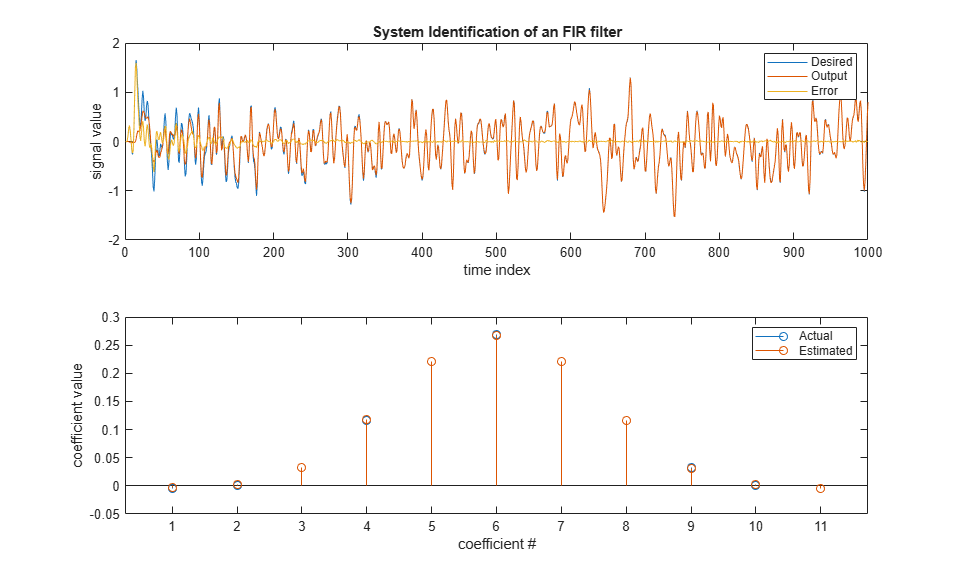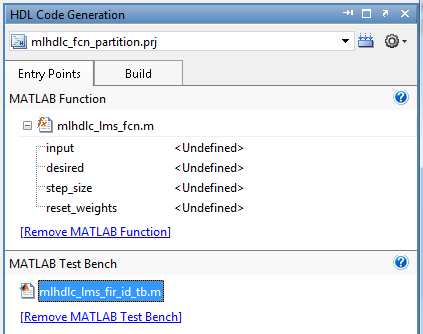# Generating Modular HDL Code for Functions

This example shows how to generate modular HDL code from MATLAB® code that contains functions.

By default, HDL Coder™ inlines the body of all MATLAB functions that are called inside the body of the top-level design function. This inlining results in the generation of a single file that contains the HDL code for the functions. To generate modular HDL code, use the Generate instantiable code for functions setting. When you enable this setting, HDL Coder generates a single VHDL® entity or Verilog® module for each function.

### LMS Filter MATLAB Design

The MATLAB design used in the example is an implementation of an LMS (Least Mean Squares) filter. The LMS filter is a class of adaptive filter that identifies an FIR filter signal that is embedded in the noise. The LMS filter design implementation in MATLAB consists of a top-level function `mlhdlc_lms_fcn` that calculates the optimal filter coefficients to reduce the difference between the output signal and the desired signal.

```design_name = 'mlhdlc_lms_fcn'; testbench_name = 'mlhdlc_lms_fir_id_tb'; ```

Review the MATLAB design:

```open(design_name); ```
```%%%%%%%%%%%%%%%%%%%%%%%%%%%%%%%%%%%%%%%%%%%%%%%%%%%%%%%%%%%%%%%%%%%%%%%%%% % MATLAB Design: Adaptive Noise Canceler algorithm using Least Mean Square % (LMS) filter implemented in MATLAB % % Key Design pattern covered in this example: % (1) Use of function calls % (2) Function inlining vs instantiation knobs available in the coder % (3) Use of system objects in the testbench to stream test vectors into the design %%%%%%%%%%%%%%%%%%%%%%%%%%%%%%%%%%%%%%%%%%%%%%%%%%%%%%%%%%%%%%%%%%%%%%%%%% %#codegen function [filtered_signal, y, fc] = mlhdlc_lms_fcn(input, ... desired, step_size, reset_weights) % 'input' : The signal from Exterior Mic which records the ambient noise. % 'desired': The signal from Pilot's Mic which includes % original music signal and the noise signal % 'err_sig': The difference between the 'desired' and the filtered 'input' % It represents the estimated music signal (output of this block) % % The LMS filter is trying to retrieve the original music signal('err_sig') % from Pilot's Mic by filtering the Exterior Mic's signal and using it to % cancel the noise in Pilot's Mic. The coefficients/weights of the filter % are updated(adapted) in real-time based on 'input' and 'err_sig'. % register filter coefficients persistent filter_coeff; if isempty(filter_coeff) filter_coeff = zeros(1, 40); end % Variable Filter: Call 'tapped_delay_fcn' function on path to create % 40-step tapped delay delayed_signal = mtapped_delay_fcn(input); % Apply filter coefficients weight_applied = delayed_signal .* filter_coeff; % Call treesum function on matlab path to sum up the results filtered_signal = mtreesum_fcn(weight_applied); % Output estimated Original Signal td = desired; tf = filtered_signal; esig = td - tf; y = esig; % Update Weights: Call 'update_weight_fcn' function on MATLAB path to % calculate the new weights updated_weight = update_weight_fcn(step_size, esig, delayed_signal, ... filter_coeff, reset_weights); % update filter coefficients register filter_coeff = updated_weight; fc = filter_coeff; function y = mtreesum_fcn(u) %Implement the 'sum' function without a for-loop % y = sum(u); % The loop based implementation of 'sum' function is not ideal for % HDL generation and results in a longer critical path. % A tree is more efficient as it results in % delay of log2(N) instead of a delay of N delay % This implementation shows how to explicitly implement the vector sum in % a tree shape to enable hardware optimizations. % The ideal way to code this generically for any length of 'u' is to use % recursion but it is not currently supported by MATLAB Coder % NOTE: To instruct MATLAB Coder to compile an external function, % add the following compilation directive or pragma to the function code %#codegen % This implementation is hardwired for a 40tap filter. level1 = vsum(u); level2 = vsum(level1); level3 = vsum(level2); level4 = vsum(level3); level5 = vsum(level4); level6 = vsum(level5); y = level6; function output = vsum(input) coder.inline('always'); vt = input(1:2:end); for i = int32(1:numel(input)/2) k = int32(i*2); vt(i) = vt(i) + input(k); end output = vt; function tap_delay = mtapped_delay_fcn(input) % The Tapped Delay function delays its input by the specified number % of sample periods, and outputs all the delayed versions in a vector % form. The output includes current input % NOTE: To instruct MATLAB Coder to compile an external function, % add the following compilation directive or pragma to the function code %#codegen persistent u_d; if isempty(u_d) u_d = zeros(1,40); end u_d = [u_d(2:40), input]; tap_delay = u_d; function weights = update_weight_fcn(step_size, err_sig, ... delayed_signal, filter_coeff, reset_weights) % This function updates the adaptive filter weights based on LMS algorithm % Copyright 2007-2022 The MathWorks, Inc. % NOTE: To instruct MATLAB Coder to compile an external function, % add the following compilation directive or pragma to the function code %#codegen step_sig = step_size .* err_sig; correction_factor = delayed_signal .* step_sig; updated_weight = correction_factor + filter_coeff; if reset_weights weights = zeros(1,40); else weights = updated_weight; end ```

The MATLAB function is modular and uses functions:

• `mtapped_delay_fcn` to calculate delayed versions of the input signal in vector form.

• `mtreesum_fcn` to calculate the sum of the applied weights in a tree structure. The individual sum is calculated by using a `vsum` function.

• `update_weight_fcn` to calculate the updated filter weights based on the least mean square algorithm.

### LMS Filter MATLAB Test Bench

Review the MATLAB test bench:

```open(testbench_name); ```
```clear ('mlhdlc_lms_fcn'); % returns an adaptive FIR filter System object, HLMS, that computes the % filtered output, filter error, and the filter weights for a given input % and desired signal using the Least MeanSquares (LMS) algorithm. % Copyright 2011-2022 The MathWorks, Inc. stepSize = 0.01; reset_weights =false; hfilt = dsp.FIRFilter; % System to be identified hfilt.Numerator = fir1(10, .25); rng('default'); % always default to known state x = randn(1000,1); % input signal d = step(hfilt, x) + 0.01*randn(1000,1); % desired signal hSrc = dsp.SignalSource(x); hDesiredSrc = dsp.SignalSource(d); hOut = dsp.SignalSink; hErr = dsp.SignalSink; %%%%%%%%%%%%%%%%%%%%%%%%%%%% %Call to the design %%%%%%%%%%%%%%%%%%%%%%%%%%%% while (~isDone(hSrc)) [y, e, w] = mlhdlc_lms_fcn(step(hSrc), step(hDesiredSrc), ... stepSize, reset_weights); step(hOut, y); step(hErr, e); end figure('Name', [mfilename, '_plot']); subplot(2,1,1), plot(1:1000, [d,hOut.Buffer,hErr.Buffer]); title('System Identification of an FIR filter'); legend('Desired', 'Output', 'Error'); xlabel('time index'); ylabel('signal value'); subplot(2,1,2); stem([hfilt.Numerator.', w(end-10:end).']); legend('Actual','Estimated'); xlabel('coefficient #'); ylabel('coefficient value'); ```

### Test the MATLAB Algorithm

To avoid run-time errors, simulate the design with the test bench.

```mlhdlc_lms_fir_id_tb ```### Create an HDL Coder Project

To generate HDL code from a MATLAB design:

1. Create a HDL Coder project:

```coder -hdlcoder -new mlhdlc_fcn_partition ```

2. Add the file `mlhdlc_lms_fcn.m` to the project as the MATLAB Function and `mlhdlc_lms_fir_id_tb.m` as the MATLAB Test Bench.

3. Click Autodefine types to use the recommended types for the inputs and outputs of the MATLAB function `mlhdlc_lms_fcn`.

Refer to Get Started with MATLAB to HDL Workflow for a more complete tutorial on creating and populating MATLAB HDL Coder projects.### Run Fixed-Point Conversion and HDL Code Generation

2. Right click the HDL Code Generation task and select Run to selected task.

A single HDL file `mlhdlc_lms_fcn_FixPt.vhd` is generated for the MATLAB design. The VHDL code for all functions in the MATLAB design is inlined into this file.

### Generate Instantiable HDL Code

1. In the Advanced tab, select the Generate instantiable code for functions check box.

2. Click the Run button to rerun the HDL Code Generation task.

You see multiple HDL files that contain the generated code for the top-level function and the functions that are called inside the top-level function. See also Generate Instantiable Code for Functions.

### Control Inlining For Each Function

In some cases, you may want to inline the HDL code for helper functions and utilities and then instantiate them. To locally control inlining of such functions, use the `coder.inline` pragma in the MATLAB code.

To inline a function in the generated code, place this directive inside that function:

```coder.inline('always') ```

To prevent inlining of a function in the generated code, place this directive inside that function:

```coder.inline('never') ```

To let the code generator determine whether to inline a function in the generated code, place this directive inside that function:

```coder.inline('default') ```

To learn how to use `coder.inline` pragma, enter:

```help coder.inline ```

### Limitations for Instantiating HDL Code from Functions

• Function calls inside conditional expressions and for loops are inlined and are not instantiated.

• Functions with states are inlined.